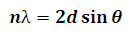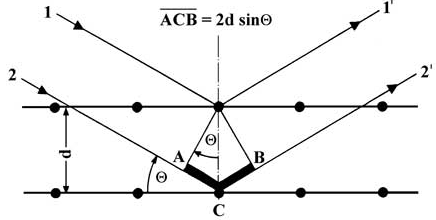# Bragg's Law Calculator

This Calctown Calculator calculates the angle of incidence at which peaks of scattered intensity are observed.

nm
nm

#### Result

degClick here to view image

where

n = integer (order of maxima or peak)

λ = wavelength of X ray

d = spacing between crystal layers

θ = incident angle